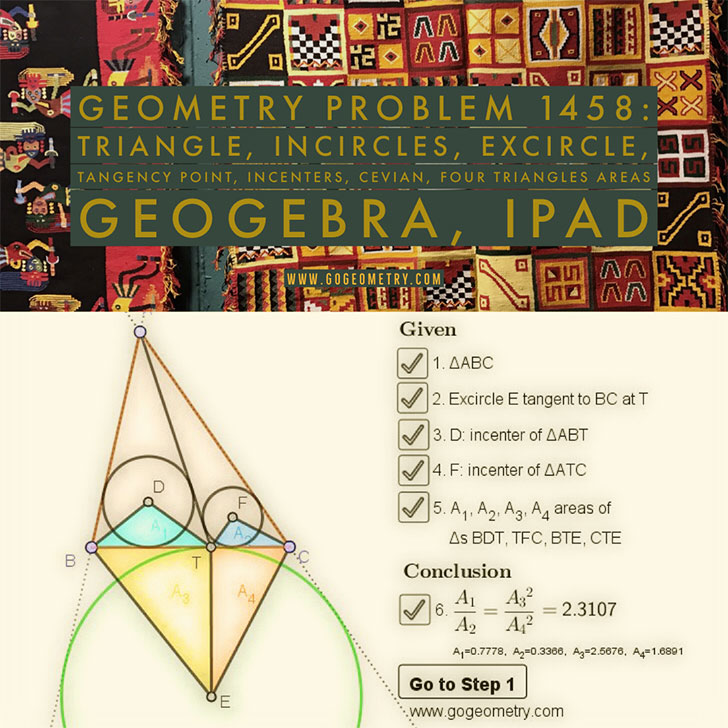# Geometry Problem 1458: Triangle, Incircles, Excircle, Area, Step-by-step Illustration

In a triangle ABC, the excircle E is tangent to the side BC at T (see the figure below). D and F are the incenters of triangles ABT and ATC. If A1, A2, A3, A4 are the areas of triangles BDT, TFC, BTE, and CTE, prove that $$\dfrac{A_{1}}{A_{2}}=\dfrac{{A_{3}}^{2}}{{A_{4}}^{2}}$$.

## Static Diagram of problem 1458## Poster of the problem 1458 using iPad Apps### Classroom Resource:Interactive step-by-step animation using GeoGebra

This step-by-step interactive illustration was created with GeoGebra.

• To explore (show / hide): click/tap a check box.
• To stop/play the animation: click/tap the icon in the lower left corner.
• To go to first step: click/tap the "Go to step 1" button.
• To manipulate the interactive figure: click/tap and drag the blue points or figures.

GeoGebra is free and multi-platform dynamic mathematics software for all levels of education that joins geometry, algebra, tables, graphing, statistics and calculus application, intended for teachers and students. Many parts of GeoGebra have been ported to HTML5.